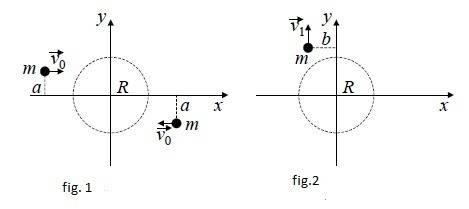# Linear and angular momentum## Homework Statement

Two atoms of equal mass m, that move with the same speed but opposite direction, interact when they're in some region R of space, as in fig.1. After the interaction, one of the atoms moves with velocity ## \vec{V1} ## as in fig.2.

a) Are the linear and angular momentum of the system conserved?
b) Calculate the velocity of the center of mass, before, during and after the interaction.
c) Find the position of the center of mass, before, during and after the interaction.
d) What is the velocity of the other atom after the interaction ?
e) Find the trajectory of the other atom after the interaction.
f) Compare ## \vec{V1} ## with ## \vec{V0} ## for different values of the impact parameter a, that is, in the cases where a>b, a=b, a<b.

## Homework Equations

## \vec{p} \equiv m\vec{v} ##

## \vec{L} = \vec{r} X \vec{p} ##

## The Attempt at a Solution

a) I thought that since there are no external forces acting on the system, both angular and linear momentums are conserved.
c) Since both atoms have the same mass and speed but opposite directions, and are at the same distance from the center O of the coordinate system, the center of mass stays there before, during and after the interaction.
b) From the above, the velocity of the center of mass equals cero at all times.
d) Since momentum must be conserved, the velocity of the other atom must be ## \vec{V} = - V1 \hat{j} ##
e) The trajectory of the other atom should be a straight line at X=b, for Y<0. Is this a correct way to put it?
f) This I don't know quite well how to put it, it seems to me that for a=b , V=V1 from simmetry, but I don't know in the other two cases.

Can anyone give me a hint? Thanks in advance.

vela
Staff Emeritus
Homework Helper
Use conservation of angular momentum.

Ok, using your hint, I thought that since a is the only component of the distance ## \vec{r} ## that's involved in the cross product, I get for the first case:

## \vec{L} = 2a m V0 ##

and using the same reasoning in the second case I get

## \vec{L}= 2bmV1 ##

Since they should be equal, I finally get a/b = V1/V0, from where I can relate the impact parameters with the velocities. Is this correct?.

vela
Staff Emeritus